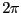Next: Distance between two angles Up: Utilities for trigonometric equation Previous: Utilities for trigonometric equation   Contents

Inclusion in an angle interval

The procedure:

int Angle_Ok_Interval(double angle,double b1,double b2);
return 1 if the angle angle belongs to the angle interval [b1,b2], 0 otherwise. The angle angle,b1, b2 should have a value within [0,].

Jean-Pierre Merlet 2012-12-20